# KR101658629B1 - Dispaly apparatus and controlling method thereof - Google Patents

## Info

Publication number
KR101658629B1
KR101658629B1 KR1020140053439A KR20140053439A KR101658629B1 KR 101658629 B1 KR101658629 B1 KR 101658629B1 KR 1020140053439 A KR1020140053439 A KR 1020140053439A KR 20140053439 A KR20140053439 A KR 20140053439A KR 101658629 B1 KR101658629 B1 KR 101658629B1
Authority
KR
South Korea
Prior art keywords
curvature
viewing
display unit
viewing angle
angle
Prior art date
Application number
KR1020140053439A
Other languages
Korean (ko)
Other versions
KR20150126172A (en
Inventor
조영귀
Original Assignee
삼성전자주식회사
Priority date (The priority date is an assumption and is not a legal conclusion. Google has not performed a legal analysis and makes no representation as to the accuracy of the date listed.)
Filing date
Publication date
Application filed by 삼성전자주식회사 filed Critical 삼성전자주식회사
Priority to KR1020140053439A priority Critical patent/KR101658629B1/en
Publication of KR20150126172A publication Critical patent/KR20150126172A/en
Application granted granted Critical
Publication of KR101658629B1 publication Critical patent/KR101658629B1/en

## Images

•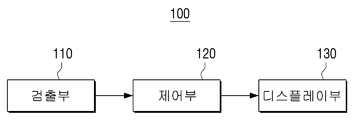•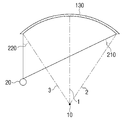••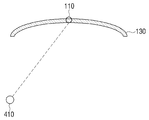•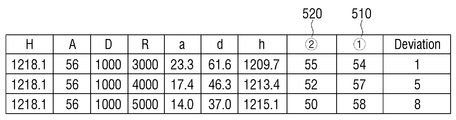•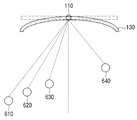•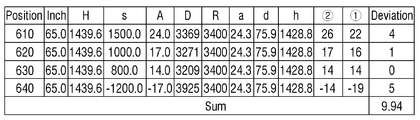••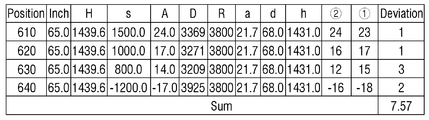•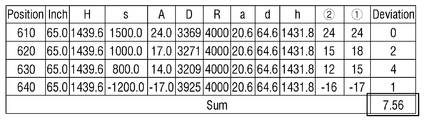•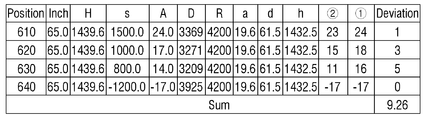••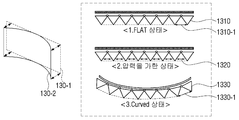•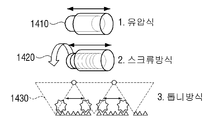•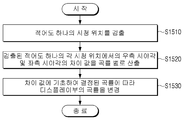••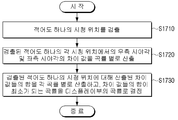## Classifications

• GPHYSICS
• G09EDUCATION; CRYPTOGRAPHY; DISPLAY; ADVERTISING; SEALS
• G09GARRANGEMENTS OR CIRCUITS FOR CONTROL OF INDICATING DEVICES USING STATIC MEANS TO PRESENT VARIABLE INFORMATION
• G09G5/00Control arrangements or circuits for visual indicators common to cathode-ray tube indicators and other visual indicators
• G09G5/14Display of multiple viewports
• GPHYSICS
• G09EDUCATION; CRYPTOGRAPHY; DISPLAY; ADVERTISING; SEALS
• G09GARRANGEMENTS OR CIRCUITS FOR CONTROL OF INDICATING DEVICES USING STATIC MEANS TO PRESENT VARIABLE INFORMATION
• G09G3/00Control arrangements or circuits, of interest only in connection with visual indicators other than cathode-ray tubes
• G09G3/001Control arrangements or circuits, of interest only in connection with visual indicators other than cathode-ray tubes using specific devices not provided for in groups G09G3/02 - G09G3/36, e.g. using an intermediate record carrier such as a film slide; Projection systems; Display of non-alphanumerical information, solely or in combination with alphanumerical information, e.g. digital display on projected diapositive as background
• G09G3/003Control arrangements or circuits, of interest only in connection with visual indicators other than cathode-ray tubes using specific devices not provided for in groups G09G3/02 - G09G3/36, e.g. using an intermediate record carrier such as a film slide; Projection systems; Display of non-alphanumerical information, solely or in combination with alphanumerical information, e.g. digital display on projected diapositive as background to produce spatial visual effects
• GPHYSICS
• G09EDUCATION; CRYPTOGRAPHY; DISPLAY; ADVERTISING; SEALS
• G09GARRANGEMENTS OR CIRCUITS FOR CONTROL OF INDICATING DEVICES USING STATIC MEANS TO PRESENT VARIABLE INFORMATION
• G09G2300/00Aspects of the constitution of display devices
• G09G2300/04Structural and physical details of display devices
• GPHYSICS
• G09EDUCATION; CRYPTOGRAPHY; DISPLAY; ADVERTISING; SEALS
• G09GARRANGEMENTS OR CIRCUITS FOR CONTROL OF INDICATING DEVICES USING STATIC MEANS TO PRESENT VARIABLE INFORMATION
• G09G2320/00Control of display operating conditions
• G09G2320/02Improving the quality of display appearance
• G09G2320/0261Improving the quality of display appearance in the context of movement of objects on the screen or movement of the observer relative to the screen
• GPHYSICS
• G09EDUCATION; CRYPTOGRAPHY; DISPLAY; ADVERTISING; SEALS
• G09GARRANGEMENTS OR CIRCUITS FOR CONTROL OF INDICATING DEVICES USING STATIC MEANS TO PRESENT VARIABLE INFORMATION
• G09G2320/00Control of display operating conditions
• G09G2320/02Improving the quality of display appearance
• G09G2320/028Improving the quality of display appearance by changing the viewing angle properties, e.g. widening the viewing angle, adapting the viewing angle to the view direction
• GPHYSICS
• G09EDUCATION; CRYPTOGRAPHY; DISPLAY; ADVERTISING; SEALS
• G09GARRANGEMENTS OR CIRCUITS FOR CONTROL OF INDICATING DEVICES USING STATIC MEANS TO PRESENT VARIABLE INFORMATION
• G09G2320/00Control of display operating conditions
• GPHYSICS
• G09EDUCATION; CRYPTOGRAPHY; DISPLAY; ADVERTISING; SEALS
• G09GARRANGEMENTS OR CIRCUITS FOR CONTROL OF INDICATING DEVICES USING STATIC MEANS TO PRESENT VARIABLE INFORMATION
• G09G2354/00Aspects of interface with display user
• GPHYSICS
• G09EDUCATION; CRYPTOGRAPHY; DISPLAY; ADVERTISING; SEALS
• G09GARRANGEMENTS OR CIRCUITS FOR CONTROL OF INDICATING DEVICES USING STATIC MEANS TO PRESENT VARIABLE INFORMATION
• G09G2380/00Specific applications
• G09G2380/02Applications of flexible displays

## Abstract

A display device is disclosed. The display device includes a detection unit for detecting at least one viewing position, a display unit capable of changing the curvature, and a difference value between the right viewing angle and the left viewing angle at each of the detected at least one viewing position by curvature, And a control unit for changing the curvature of the display unit according to the determined curvature. Accordingly, the optimum curvature for reducing the viewing angle distortion is determined in consideration of the viewing position of at least one viewer, and the curvature of the display unit can be changed according to the determined optimal curvature.

## Description

 DISPLAY APPARATUS AND CONTROLLING METHOD THEREOF 

BACKGROUND OF THE INVENTION 1. Field of the Invention  The present invention relates to a display apparatus and a control method thereof, and more particularly, to a display apparatus and a control method thereof for changing a curvature of a display unit.

Various types of electronic products are being developed and distributed by the development of electronic technology. In particular, various display devices such as TVs, mobile phones, PCs, notebook PCs, and PDAs are used in most households.

As the use of display devices has increased, the user needs for more diverse functions have also increased. As a result, the effort of each manufacturer to meet user needs has increased, and products with new functions that have not been available in the past are emerging.

Particularly, a display device in which a left / right curved display portion of the display portion has a larger audiovisual angle than a flat-type display device can increase the immersion feeling.

However, in the case of a viewer located on the side of a display device with a left / right curved display portion, distortion of the viewing angle is increased and a distorted image is viewed.

Accordingly, there has been a growing demand for changing the curvature of the display unit in accordance with the obtained optimum radius of curvature to obtain an optimum radius of curvature that minimizes the problem of viewing angle distortion, considering the position of at least one viewer.

It is an object of the present invention to provide a display device and a control method thereof for changing a curvature of a display portion in order to reduce viewing angle distortion.

According to an aspect of the present invention, there is provided a display apparatus including a detection unit detecting at least one viewing position, a display unit capable of changing a curvature, And a control unit for calculating a difference value of the viewing angle for each curvature and changing the curvature of the display unit according to the curvature determined based on the difference value.

Here, the controller determines a curvature at which the difference value is minimized as a curvature of the display unit.

The control unit calculates a sum of difference values calculated for the detected at least one viewing position for each curvature, and determines a curvature at which the sum of the difference values becomes minimum as a curvature of the display unit.

The control unit sets the curvature of the display unit to the first curvature and calculates the difference between the right viewing angle and the left viewing angle in each of the detected at least one viewing position and sets the curvature of the display unit to the second curvature And calculates a difference value between the right viewing angle and the left viewing angle in each of the detected at least one viewing position.

The control unit calculates an audiovisual angle of each of the viewing positions based on the viewing direction from each of the detected at least one viewing position, and calculates a viewing angle of each of the detected at least one viewing position and a plane And calculates the difference between the right viewing angle and the left viewing angle at each viewing position based on the calculated viewing angle and viewing distance.

Meanwhile, the audiovisual angle can be calculated through Equation (1).

Here, S is the length in the horizontal direction from the viewing position to the detection unit, and D is the viewing distance.

D is the viewing distance, A is the audiovisual angle, h is the horizontal straight line length of the display unit, d is the viewing angle, and d is the viewing angle. The right viewing angle is calculated using Equation 2 and the left viewing angle is calculated through Equation Represents the thickness of the display portion, and a represents the curvature angle.

In addition, the sum of the difference values calculated for the detected at least one viewing position is calculated according to Equation (7) for each curvature.

Here, the display unit may include a plurality of blocks spaced apart from each other, and the controller may change a distance between adjacent blocks by applying a pressure corresponding to the determined curvature to the plurality of blocks to adjust a curvature of the display unit Can be changed.

Meanwhile, a method of controlling a display device according to an embodiment of the present invention includes detecting at least one viewing position, calculating a difference value between a right viewing angle and a left viewing angle at each of the detected at least one viewing position by curvature And changing the curvature of the display portion according to the curvature determined based on the difference value.

Here, the changing step may determine the curvature at which the difference value is minimized as the curvature of the display unit.

The changing step calculates the sum of difference values calculated for the detected at least one viewing position for each curvature, and determines the curvature at which the sum of the difference values becomes minimum as the curvature of the display unit.

The calculating step may include calculating a difference between a right viewing angle and a left viewing angle in each of the detected at least one viewing position by setting a curvature of the display part to a first curvature and changing a curvature of the display part to a second curvature And calculates a difference value between the right viewing angle and the left viewing angle in each of the detected at least one viewing position.

The calculating step may include calculating an audiovisual angle of each of the viewing positions based on a viewing direction from each of the detected at least one viewing position and calculating a viewing angle of each of the detected at least one viewing position and a plane And calculates the difference between the right viewing angle and the left viewing angle at each viewing position based on the calculated viewing angle and viewing distance.

On the other hand, the audiovisual angle is calculated through Equation (1).

Here, S is the length in the horizontal direction from the viewing position to the detection unit, and D is the viewing distance.

The right viewing angle is calculated using Equation 2, and the left viewing angle is calculated using Equation 3, where D is the viewing distance, A is the audiovisual angle, h is the horizontal straight line length of the display portion, d Represents the thickness of the display portion, and a represents the curvature angle.

In addition, the sum of the difference values calculated for the detected at least one viewing position is calculated by the following Equation (7) for each curvature.

Here, the changing step may change a curvature of the display unit by changing a distance between adjacent blocks by applying a pressure corresponding to the determined curvature to a plurality of blocks included in the display unit.

According to various embodiments of the present invention as described above, the optimum curvature for reducing the viewing angle distortion is determined in consideration of the viewing position of at least one viewer, and the curvature of the display unit can be changed according to the determined optimal curvature.

1 is a block diagram illustrating a configuration of a display apparatus according to an exemplary embodiment of the present invention.
2 is a view illustrating a right viewing angle and a left viewing angle required to set the curvature of the display unit according to an embodiment of the present invention.
3 is a view illustrating information necessary for setting a curvature of a display unit according to an exemplary embodiment of the present invention.
4 and 5 are views for explaining a process of determining a curvature for one viewing position according to an embodiment of the present invention.
6 to 12 are diagrams for explaining a process of determining a curvature in consideration of a plurality of viewing positions according to an embodiment of the present invention.
13 is a diagram illustrating a configuration of a display unit according to an embodiment of the present invention.
Figure 14 is a diagram of a method of applying pressure in accordance with one embodiment of the present invention.
15 is a flowchart illustrating a method of controlling a display device according to an embodiment of the present invention.
16 is a flowchart illustrating a method of determining a curvature of a display unit according to an embodiment of the present invention.
17 is a flowchart illustrating a method of determining a curvature of a display unit according to another embodiment of the present invention.

Hereinafter, the present invention will be described in more detail with reference to the drawings. In the following description, a detailed description of known functions and configurations incorporated herein will be omitted when it may make the subject matter of the present invention rather unclear. Hereinafter, the terms are defined in consideration of the functions of the present invention, which may vary depending on the intention or the relationship of the user, the operator, and the like. Therefore, the definition should be based on the contents throughout this specification.

1 is a block diagram illustrating a configuration of a display apparatus according to an exemplary embodiment of the present invention.

1, the display device 100 includes a detection unit 110, a control unit 120, and a display unit 130. [ Here, the display device 100 may be implemented as various types of electronic devices such as a TV, an electronic whiteboard, an electronic table, a large format display (LFD), a smart phone, a tablet, a desktop PC,

The detection unit 110 can detect at least one viewing position. Here, the viewing position refers to the position of a viewer who watches the display device 100. Specifically, the detection unit 110 may be implemented by a camera, an IR sensor, or the like.

When the detection unit 110 is implemented by a camera, the detection unit 110 can detect the viewing position of the viewer based on the focal distance when capturing the viewer. When the detection unit 110 is implemented as an IR sensor, the detection unit 110 can detect a viewing position of a viewer based on a region where infrared rays are reflected, a reception time of infrared rays, and the like.

The detection unit 110 may be disposed at any one of the corners, the upper and lower ends of the display device 100, the detection unit 110 may be disposed, or a plurality of the detection units 110 may be disposed .

The display unit 130 has a predetermined curvature or curvature radius by bending the left and right sides of the display unit in a planar state. Here, the radius of curvature or radius of curvature is a value capable of indicating the degree of curvature at each point on the curved surface. That is, as the radius of curvature or radius of curvature becomes larger, the curvature of the curved surface becomes gentle, and as the radius of curvature or radius of curvature becomes smaller, the curvature of the curved surface becomes sharper.

In the present specification, the curvature and the radius of curvature are all defined to mean a value capable of indicating the degree of curvature at each point of the curved surface.

In addition, the display unit 130 may include a display panel (not shown) and a backlight unit (not shown), and the display panel (not shown) and the backlight unit (not shown) That is, when the display unit 130 is varied according to the determined curvature, the display panel (not shown) and the backlight unit (not shown) may also vary according to the determined curvature.

The control unit 120 may calculate the difference between the right viewing angle and the left viewing angle at each detected viewing position for each curvature and change the curvature of the display unit 130 according to the curvature determined based on the difference.

In other words, a user located at the center of the display unit 130 has a greater viewing angle than the flat display unit, A distortion of the viewing angle occurs.

In order to reduce the distortion of the viewing angle, the controller 120 calculates a difference value between the right viewing angle and the left viewing angle at each of the at least one viewing position detected by the detecting unit 110 for each curvature.

2 is a view showing a right viewing angle and a left viewing angle required to set the curvature of the display unit according to an embodiment of the present invention.

Referring to FIG. 2, the display unit 130 may be bent to have a predetermined curvature so that the display unit 130 may correspond to a part of a circle having one center 10. A line 2 positioned in a direction perpendicular to the tangent of the right end of the display unit 130 and a line 2 located in a direction perpendicular to the tangent of the display area 130 The line 3 positioned in a direction perpendicular to the tangent line of the left end of the display unit 130 is a radius having the same length and all meet at the center point 10 of the circle including the display unit 130. [

In the viewing position 20 at which the user is located, the angle between the line of sight of the right end of the display unit 130 and the line 2 of the line perpendicular to the tangent of the right end of the display unit 130 A line 3 that is positioned in a direction perpendicular to the tangent line between the left end of the display unit 130 and the line of sight of the left end of the display unit 130 in the viewing position 20 where the user is located, ) Is the left viewing angle.

The viewing direction in which the user views the right end of the display unit 130 in the viewing position 20 and the viewing direction in which the left end of the display unit 130 is viewed in the viewing position 20 in which the user is located, 110).

The control unit 120 calculates a difference value between the right viewing angle 210 and the left viewing angle 220 in the viewing position 20 where the user is located. The smaller the difference between the right viewing angle 210 and the left viewing angle 220 in the viewing position 20 where the user is located, the smaller the viewing angle distortion in the viewing position 20 where the user is located.

The control unit 120 may repeatedly calculate the difference between the right viewing angle and the left viewing angle at each of the at least one viewing position detected by the detecting unit 110 for each curvature.

Specifically, the controller 120 sets the curvature of the display unit 130 to the first curvature, and calculates the difference between the right viewing angle and the left viewing angle at each of the detected viewing positions.

For example, the control unit 120 may set the curvature of the display unit 130 to 3000R, and calculate the difference between the right viewing angle and the left viewing angle at the detected at least one viewing position.

Then, the control unit 120 sets the curvature of the display unit 130 to the second curvature, and calculates the difference between the right viewing angle and the left viewing angle at each of the detected viewing positions.

That is, the control unit 120 may set the curvature of the display unit 130 to 4000R, and calculate the difference between the right viewing angle and the left viewing angle at the detected at least one viewing position.

On the other hand, the control unit 120 may calculate a difference value between the right viewing angle and the left viewing angle at the changed viewing position.

For example, when the user watches while moving in front of the display device 100, the detecting unit 110 can detect that the viewing position of the user is moving and continuously detect the changed viewing position of the user. Accordingly, the control unit 120 can calculate the difference value between the right viewing angle and the left viewing angle at each viewing position based on the change of the viewing position continuously detected by the detecting unit 110. [ That is, the control unit 120 can calculate the difference value between the right viewing angle and the left viewing angle at each viewing position in real time according to the movement or change of the viewing position.

Also in this case, the control unit 120 can calculate the difference value between the right viewing angle and the left viewing angle at each viewing position in real time for each curvature.

As described above, the control unit 120 can calculate the difference value between the right viewing angle and the left viewing angle based on various curvatures of the display unit 130 and various detected viewing positions.

Here, a detailed process of calculating the difference value between the right viewing angle and the left viewing angle at the viewing position will be described in detail.

3 is a view illustrating information necessary for setting a curvature of a display unit according to an exemplary embodiment of the present invention.

3, the display unit 130, the right viewing angle 210, the left viewing angle 220, the thickness 230 of the display unit 130, the viewing distance 240, the curvature angle 250 A horizontal line length 270 of the display unit 130 and a horizontal length 280 from the detected viewing position 20 to the detection unit 110 are shown.

2, the right viewing angle 210 corresponds to the viewing direction 4 in which the right end of the display unit 130 is viewed from the viewing position 20 detected by the detecting unit 110, And the left viewing angle 220 is an angle formed by the line 2 positioned in a direction perpendicular to the tangent of the right end of the display unit 130 and the left viewing angle 220, And an angle formed by the line of sight 6 and the line 3 located in a direction perpendicular to the tangent line of the left end of the display unit 130. [

On the other hand, the control unit 120 can calculate the audiovisual angle of each viewing position based on the viewing direction from each of the detected at least one viewing position.

3, the controller 120 controls the viewing direction 5 in which the detection unit 110 is viewed from the detected viewing position 20 and the viewing direction 5 in the direction perpendicular to the tangent of the region in which the detection unit 110 exists It is possible to calculate the audiovisual angle 260 in the detected viewing position 20 by comparing the virtual line 1 positioned.

Information about the virtual line 1 located in a direction perpendicular to the tangent line of the region where the detection unit 110 exists is stored in a storage unit (not shown), and the control unit 120 stores information Information about a virtual line 1 located in a direction perpendicular to the tangent of the region where the detection unit 110 stored in the detection unit 110 exists and information about the virtual line 1 located in the direction of the detection unit 110 from the viewing position 20 detected by the detection unit 110 And calculates the audiovisual angle 260 based on the information on the viewing direction 5.

In addition, the control unit 120 can calculate the viewing distance of each viewing position, which is a straight line connecting the detected at least one viewing position and the plane on which the detecting unit 110 is located.

3, the control unit 120 may calculate the viewing distance 240 based on the straight line distance between the plane on which the detecting unit 110 is located and the detected viewing position 20 vertically.

For example, if the detection unit 110 is a camera, it is assumed that there is a lookup table storing information on the viewing distance 240 corresponding to each focal length. The viewing distance 240 may be calculated by obtaining information on the viewing distance 240 corresponding to the focal length from the lookup table.

On the other hand, the control unit 120 can calculate the difference value between the right viewing angle and the left viewing angle at each viewing position based on the calculated viewing angle and viewing distance.

In particular, the audiovisual angle 260 can be calculated through the following equation.

Here, S represents the length 280 in the horizontal direction from the viewing position to the detection unit 110, and D represents the viewing distance 240. [

That is, the audiovisual angle 260 can be changed by the detected viewing position 20 and viewing distance 240. Here, the control unit 120 stores information on the virtual line 1 positioned in the direction perpendicular to the tangent of the area where the detection unit 110 is stored, and information on the detected viewing position 20 in the storage unit (not shown) The horizontal length 280 from the viewing position to the detection unit 110 can be calculated.

On the other hand, the right viewing angle 210 can be calculated by the following equation.

The left viewing angle 220 can be calculated by the following equation.

Where d is the viewing distance 240, A is the audiovisual angle 260, h is the horizontal straight line length 270 of the display 130, d is the thickness of the display 130, a is the curvature angle 250, .

A curvature angle 250 is defined as a line 2 connecting the center point 10 of the circle corresponding to the curvature of the display unit 130 and the right end of the display unit 130, And the line 3 connecting the center point 10 of the circle corresponding to the display unit 130 and the left end of the display unit 130. The curvature angle 250 can be calculated by the following equation.

Here, H denotes an actual horizontal length of the display unit 130. That is, the actual horizontal length of the curved display unit 130 is the same as the length of the arc that is a part of the circle corresponding to the curvature of the display unit 130.

R denotes the radius of a circle corresponding to the curvature of the display unit 130, which is the same as the distance from the center point 10 of the circle to the display unit 130. It is also the same as the radius of curvature.

If a value obtained by dividing the actual horizontal length of the display unit 130 by the radius of curvature is represented by rad, that is, by the arithmetic method, the curvature angle 250 can be calculated.

On the other hand, the thickness 230 of the display unit 130 can be calculated by the following equation.

The controller 120 may determine the thickness 230 of the display unit 130 using Equation 5 using the curvature radius 250 and the curvature radius R obtained from Equation (4).

The straight line distance 270 in the horizontal direction of the display unit 130, h, can be calculated by the following equation.

The straight distance 270 in the horizontal direction of the display unit 130 refers to the straight line distance between the left and right ends of the display unit 130 and is different from the actual horizontal length of the display unit 130 described above.

The controller 120 calculates the right and left viewing angles at the respective viewing positions detected through Equations 2 and 3 and calculates the difference between the calculated right viewing angle and left viewing angle.

Then, the control unit 120 can determine the curvature based on the difference value. Here, the curvature determined based on the difference value means a curvature capable of minimizing the viewing angle distortion. Specifically, the controller 120 can determine the curvature at which the difference value becomes minimum as the curvature of the display unit 130. Will be described with reference to Figs. 4 and 5. Fig.

4 and 5 are views for explaining a process of determining a curvature for one viewing position according to an embodiment of the present invention.

Referring to FIG. 4, a detection unit 110 attached to the display unit 130 and the display unit 130 is illustrated, and the detection unit 110 can detect the viewing position 410 of the viewer. The control unit 120 calculates the viewing angle and viewing distance of the detected viewing position 410 and calculates the difference between the right viewing angle and the left viewing angle with respect to the detected viewing position based on the calculated viewing angle and viewing distance . The controller 120 may calculate the difference between the right viewing angle and the left viewing angle with respect to the viewing position detected according to various curvatures of the display unit 130. [

5 shows a table in which the difference between the right viewing angle and the left viewing angle calculated on the assumption that the size of the display unit 130 is 55 inches and the curvatures are 3000R, 4000R, and 5000R.

Specifically, H is the actual horizontal length of the display unit 130, A is the audiovisual angle 260, D is the viewing distance 240, R is the curvature or radius of curvature (1,2,3) D represents the thickness 230 of the display unit 130 and h represents the horizontal distance 270 of the display unit 130. Reference numeral 510 represents a right viewing angle 210, And the deviation means a difference value between the right viewing angle 210 and the left viewing angle 220 which are difference values between the first and second viewing angles.

Deviation is 1 when the curvature is 3000R, is 5 when it is 4000R, and is 8 when it is 5000R, so that the controller 120 can determine the curvature of 3000R, which is the curvature of the case where the difference value is minimized to 1, as the curvature of the display portion.

According to another embodiment, the controller 120 may calculate the sum of the calculated difference values for the detected at least one viewing position for each curvature, and display the curvature at which the sum of the difference values becomes minimum, As shown in FIG.

That is, when a plurality of viewers view at a plurality of viewing positions, in order to minimize the distortion of the viewing angle at a plurality of viewing positions, the control unit 120 calculates the sum of the calculated difference values for the plurality of viewing positions for each curvature Thereby setting the curvature that minimizes the sum of the difference values to the optimal curvature of the display unit 130. [

Here, the sum of the difference values calculated for the detected plurality of viewing positions can be calculated for each curvature through the following equation.

Here, p denotes each of a plurality of viewing positions, and according to Equation (7), the difference values of the right viewing angle and the left viewing angle with respect to each of the plurality of viewing positions are added together. Then, the controller 120 repeatedly sums the difference between the right viewing angle and the left viewing angle for each of the plurality of viewing positions by Equation (7) for each curvature.

When the +/- sign is used to distinguish the viewing position existing in the right region from the viewing position existing in the left region with respect to the detection unit 110, ABS is used to consider only the scalar amount excluding the sign .

Thus, according to the curvature that minimizes the sum of the difference values, the distortion of the viewing angle at a plurality of viewing positions existing in a predetermined area can be minimized. Specifically, this will be described with reference to Figs. 6 to 12. Fig.

6 to 12 are diagrams for explaining a process of determining a curvature in consideration of a plurality of viewing positions according to an embodiment of the present invention.

6, a detection unit 110 attached to the display unit 130 and the display unit 130 is shown. The detection unit 110 detects a plurality of viewing positions 610, 620, 630, and 640 of a plurality of viewers Can be detected. The control unit 120 calculates an audiovisual angle and a viewing distance for each of the plurality of detected viewing positions 610, 620, 630, and 640, calculates a plurality of viewing positions based on the calculated viewing angle and viewing distance, The difference between the right viewing angle and the left viewing angle can be calculated. The controller 120 may calculate the difference between the right viewing angle and the left viewing angle with respect to the viewing position detected according to various curvatures of the display unit 130. [

7 shows a table in which the difference between the right viewing angle and the left viewing angle in the plurality of viewing positions 610, 620, 630, and 640 is arranged when the size of the display unit 130 is 65 inches and the curvature is 3400R .

Specifically, since H, A, D, R, a, d, h, 1, and 2 have been described with reference to FIG. 5, a detailed description thereof will be omitted. Here, s means the length 280 in the horizontal direction from the viewing position 20 to the detection unit 110 in Fig. In FIG. 7, it can be seen that the horizontal length 280 from the plurality of viewing positions 610, 620, 630, and 640 to the detection unit 110 is set to 1500, 1000, 800, and -1200. Here, positive numbers 1500, 1000, and 800 are when the viewing position is in the left area with respect to the center area of the display part 130, and -1200 with a negative sign is in the center area of the display part 130 It means when the viewing position is in the right area as a reference.

In addition, the viewing angle of each of the plurality of viewing positions 610, 620, 630, and 640 and the length in the horizontal direction from each viewing position to the detecting unit 110 can be calculated by the controller 120.

7, when the size of the display unit 130 is 65 inches and the curvature is 3400R, it can be seen that the sum of the difference values at the plurality of viewing positions 610, 620, 630, and 640 is 9.94 have.

8 illustrates a table in which the difference between the right viewing angle and the left viewing angle in the plurality of viewing positions 610, 620, 630, and 640 is arranged when the size of the display unit 130 is 65 inches and the curvature is 3600R Referring to FIG. 8, it can be seen that the sum of the difference values at the plurality of viewing positions 610, 620, 630, and 640 is 7.58.

Likewise, Figs. 9 to 12 show a right viewing angle and a right viewing angle in a plurality of viewing positions 610, 620, 630, and 640 when the size of the display unit 130 is 65 inches and the curvatures are 3800R, 4000R, 4200R, 9 to 12, the sum of the difference values at the plurality of viewing positions 610, 620, 630, and 640 is 7.57, 7.56, 9.26, 12.02.

Therefore, the smallest value of the sum of difference values at the plurality of viewing positions 610, 620, 630, and 640 shown in FIGS. 7 to 12 is 7.56, and the curvature at this time is 4000R.

Accordingly, the controller 120 can determine the curvature of the display unit 130 to be 4000R, which is the curvature of the case where the sum of the difference values is 7.56.

In the above example, the control unit 120 calculates the sum of the difference values to determine the curvature of the display unit 130. However, the average of the difference values may be calculated, Or the curvature of the display 130 may be determined.

In the above-described example, the difference between the right viewing angle and the left viewing angle at the at least one viewing position where the controller 120 is fixed is calculated. However, the controller 120 may calculate the difference between the right viewing angle at the at least one viewing position And a difference value of the left viewing angle may be calculated.

For example, when a viewer watches while moving in front of the display device 100, the detecting unit 110 can continuously detect the viewing position of the user who changes the viewing position of the user, and accordingly, 120 can calculate the difference value between the right viewing angle and the left viewing angle at each viewing position based on the change of the viewing position continuously detected from the detecting unit 110. [

That is, the control unit 120 can calculate the difference value between the right viewing angle and the left viewing angle at each viewing position in real time according to the movement or change of the viewing position.

Also in this case, the control unit 120 can calculate the difference value between the right viewing angle and the left viewing angle at each viewing position in real time for each curvature.

Accordingly, the control unit 120 can change the curvature of the display unit 130 in real time based on the movement or the change of the viewing position.

Meanwhile, according to another embodiment, the control unit 120 may calculate a difference value between a right viewing angle and a left viewing angle at at least one viewing position with respect to the flat display unit, The difference between the right viewing angle and the left viewing angle at at least one viewing position with respect to the flat display unit and the difference between the right viewing angle at the at least one viewing position with respect to the curved display unit and the difference between the right viewing angle and the left viewing angle with respect to the curved display unit, And the curvature of the display unit 130 may be determined based on the difference values.

That is, the control unit 120 determines the difference between the difference between the right viewing angle and the left viewing angle at at least one viewing position with respect to the flat display unit and the difference between the right viewing angle and the left viewing angle at at least one viewing position with respect to the curved display unit The curvature of the display portion 130 can be determined.

Meanwhile, the control unit 120 may change the curvature of the display unit 130 according to the determined curvature. Specifically, the control unit 120 may change the curvature by bending the display unit 130 according to the curvature determined according to the above-described embodiment, that is, the optimum curvature.

The display unit 130 includes a plurality of blocks spaced apart from each other. The controller 120 applies a pressure corresponding to a curvature determined for the plurality of blocks to change a distance between adjacent blocks, The curvature can be changed.

13 is a diagram illustrating a configuration of a display unit according to an embodiment of the present invention.

Referring to FIG. 13, the display unit 130 may be bent from the flat state 130-1 to the curved state 130-2.

Specifically, the display unit 130 includes a plurality of blocks 1310 spaced apart from each other by a predetermined interval 1310-1, and the control unit 120 may apply pressure 1320 between each of the plurality of blocks. .

Here, the plurality of blocks 1310 are triangular in cross section and share one vertex. Accordingly, when the control unit 120 applies the pressure 1320 between each of the plurality of blocks, the interval 1330-1 between the plurality of pressure-receiving units 1330 is increased, Block 1330 may be curved.

According to the above-described example, the control unit 120 can change the curvature of the display unit 130 by applying a pressure of a size corresponding to the determined curvature between each of the plurality of blocks.

Figure 14 is a diagram of a method of applying pressure in accordance with one embodiment of the present invention.

Referring to Fig. 14, the pressure 1320 applied between each of the plurality of blocks can be generated by the hydraulic system 1410 or the screw system 1420 or the saw tooth system 1430.

The hydraulic system 1410 is a system in which the pressure 1320 applied between each of the plurality of blocks is adjusted in accordance with the amount of oil, water, or air introduced into each of the plurality of blocks, and the screw system 1420 controls the motor And the pressure 1320 applied between each of the plurality of blocks is adjusted according to the amount of rotation of the motor. In the saw tooth method 1430, a chain is attached to the teeth provided between each of the plurality of blocks, And the pressure 1320 applied between each of the plurality of blocks is adjusted.

On the other hand, generation of the pressure 1320 applied between each of the plurality of blocks is not limited to the above-described embodiment.

The display unit 130 includes a plurality of small motors and the control unit 120 controls the plurality of small motors to bend the left and right sides of the display unit 130 to change the curvature of the display unit 130 It is possible.

15 is a flowchart illustrating a method of controlling a display device according to an embodiment of the present invention.

According to the method shown in Fig. 15, at least one viewing position can be detected (S1510).

Thereafter, a difference value between the right viewing angle and the left viewing angle at each of the detected at least one viewing position can be calculated for each curvature (S1520).

Here, the calculating step may include calculating the difference between the right viewing angle and the left viewing angle in each of the at least one viewing position detected by setting the curvature of the display unit to the first curvature, setting the curvature of the display unit to the second curvature, The difference between the right viewing angle and the left viewing angle in each of at least one viewing position can be calculated.

The calculating step may calculate an audiovisual angle of each of the viewing positions based on the viewing direction from each of the detected at least one of the viewing positions, The viewing distances of the connected viewing positions are calculated and the difference value between the right viewing angle and the left viewing angle at each viewing position can be calculated based on the calculated viewing angle and viewing distance.

Here, the audiovisual angle is calculated through Equation (1), where S is the length in the horizontal direction from the viewing position to the detection unit, and D is the viewing distance.

The right viewing angle is calculated through Equation 2 and the left viewing angle is calculated through Equation 3 where D is the viewing distance, A is the viewing angle, h is the horizontal straight line length of the display portion, d is the thickness of the display portion, a represents the curvature angle.

Then, the curvature of the display unit can be changed according to the curvature determined based on the difference value (S1530).

Here, in the changing step, the curvature at which the difference value becomes minimum can be determined as the curvature of the display portion.

Also, the changing step may calculate the sum of the calculated difference values for at least one of the detected viewing positions for each curvature, and determine the curvature of the display unit to be the minimum of the sum of the difference values.

Here, the sum of the difference values calculated for the detected at least one viewing position may be calculated from equation (7) for each curvature.

In addition, the changing step may change the curvature of the display unit by changing the distance between adjacent blocks by applying a pressure corresponding to the curvature determined for the plurality of blocks included in the display unit.

16 is a flowchart illustrating a method of determining a curvature of a display unit according to an embodiment of the present invention.

According to the method shown in Fig. 16, at least one viewing position is detected (S1610).

Then, a difference value between the right viewing angle and the left viewing angle at each detected at least one viewing position is calculated for each curvature (S1620).

Then, the curvature at which the difference value becomes minimum is determined as the curvature of the display portion (S1630).

17 is a flowchart illustrating a method of determining a curvature of a display unit according to another embodiment of the present invention.

According to the method shown in Fig. 17, at least one viewing position is detected (S1710).

Thereafter, a difference value between the right viewing angle and the left viewing angle at each detected at least one viewing position is calculated for each curvature (S1720).

Then, the sum of the calculated difference values for at least one viewing position is calculated for each curvature, and the curvature at which the sum of the difference values becomes minimum is determined as the curvature of the display unit (S1730).

Meanwhile, a non-transitory computer readable medium having a program for sequentially performing the control method according to the present invention may be provided.

In one example, the step of detecting at least one viewing position, calculating a difference value of the right viewing angle and the left viewing angle at each detected viewing position by curvature is stored in a non-transitory readable medium (non a transitory computer readable medium may be provided.

A non-transitory readable medium is a medium that stores data for a short period of time, such as a register, cache, memory, etc., but semi-permanently stores data and is readable by the apparatus. In particular, the various applications or programs described above may be stored on non-volatile readable media such as CD, DVD, hard disk, Blu-ray disk, USB, memory card, ROM,

In addition, although a bus is not shown in the above-described block diagram of the display device, the communication between the respective components in the display device may be performed through a bus. Further, each device may further include a processor such as a CPU, a microprocessor, or the like that performs the various steps described above.

While the present invention has been particularly shown and described with reference to exemplary embodiments thereof, it is to be understood that the invention is not limited to the disclosed exemplary embodiments, but, on the contrary, It will be understood by those skilled in the art that various changes in form and detail may be made therein without departing from the spirit and scope of the present invention.

100: Display device 110: Detector
120: control unit 130:

## Claims (18)

1. A detecting unit detecting at least one viewing position;
A display unit capable of changing the curvature;
And a controller for calculating a difference value between the right viewing angle and the left viewing angle at each of the detected viewing positions by curvature and changing a curvature of the display unit according to a curvature determined based on the difference value,
Wherein the right viewing angle is an angle formed by a line positioned in a direction perpendicular to a tangent of a right end of the display unit and a line of sight of the display unit facing the right end of the display unit at the viewing position, And a line that is located in a line perpendicular to the tangent line of the left end of the display unit.
2. The method according to claim 1,
Wherein,
And determines a curvature at which the difference value becomes minimum as a curvature of the display unit.
3. The method according to claim 1,
Wherein,
Calculates a sum of difference values calculated for the detected at least one viewing position for each curvature, and determines a curvature at which a sum of the difference values becomes minimum as a curvature of the display unit.
4. The method according to claim 1,
Wherein,
Setting a curvature of the display unit to a first curvature and calculating a difference value between a right viewing angle and a left viewing angle in each of the detected at least one viewing position,
And sets a curvature of the display unit to a second curvature to calculate a difference value between a right viewing angle and a left viewing angle in each of the detected at least one viewing position.
5. 5. The method of claim 4,
Wherein,
Calculating a viewing angle of each of the viewing positions based on a viewing direction of the detected viewing position from each of the detected at least one viewing position, And calculates the difference between the right viewing angle and the left viewing angle at each of the viewing positions based on the calculated viewing angle and viewing distance.
6. 6. The method of claim 5,
Wherein the viewing angle is calculated by the following equation.

Here, S is the length in the horizontal direction from the viewing position to the detection unit, and D is the viewing distance.
7. The method according to claim 1,
The right-

, &Lt; / RTI &gt;
The left-

Where D is the viewing distance, A is the viewing angle, h is the horizontal straight line length of the display unit, d is the thickness of the display unit, and a is the curvature angle.
8. The method of claim 3,
Wherein the sum of difference values calculated for the detected at least one viewing position is calculated by the following equation for each curvature.
9. The method according to claim 1,
The display unit includes:
A plurality of blocks spaced apart from each other,
Wherein,
Wherein a curvature of the display unit is changed by changing a distance between adjacent blocks by applying a pressure corresponding to the determined curvature to the plurality of blocks.
10. Detecting at least one viewing position;
Calculating a difference value of the right viewing angle and the left viewing angle at each of the detected at least one viewing position by curvature; And
And changing the curvature of the display portion according to the curvature determined based on the difference value,
Wherein the right viewing angle is an angle formed by a line positioned in a direction perpendicular to a tangent of a right end of the display unit and a line of sight of the display unit facing the right end of the display unit at the viewing position, And a line that is located in a line perpendicular to a tangent line of the left end of the display unit and a line of sight of the left end of the display unit.
11. 11. The method of claim 10,
Wherein the modifying comprises:
And determines a curvature at which the difference value becomes minimum as a curvature of the display unit.
12. 11. The method of claim 10,
Wherein the modifying comprises:
Calculating a curvature at which the sum of difference values calculated for the detected at least one viewing position is calculated for each curvature and a curvature at which a sum of the difference values is minimized as a curvature of the display unit .
13. 11. The method of claim 10,
Wherein the calculating step comprises:
Setting a curvature of the display unit to a first curvature and calculating a difference value between a right viewing angle and a left viewing angle in each of the detected at least one viewing position,
And sets the curvature of the display unit to a second curvature to calculate a difference value between a right viewing angle and a left viewing angle in each of the detected at least one viewing position.
14. 14. The method of claim 13,
Wherein the calculating step comprises:
The viewing angle of each of the viewing positions is calculated on the basis of the viewing direction for viewing the detecting unit for detecting the viewing position from each of the detected at least one viewing position, The viewing distance of each of the viewing positions obtained by connecting the planes in a straight line perpendicularly is calculated and the difference value between the right viewing angle and the left viewing angle at each viewing position is calculated based on the calculated viewing angle and viewing distance Of the display device.
15. 15. The method of claim 14,
Wherein the audiovisual angle is calculated by the following equation.

Here, S is the length in the horizontal direction from the viewing position to the detection unit, and D is the viewing distance.
16. 11. The method of claim 10,
The right-

, &Lt; / RTI &gt;
The left-

Where D is the viewing distance, A is the audiovisual angle, h is the horizontal straight line length of the display unit, d is the thickness of the display unit, and a is the curvature angle.
17. 13. The method of claim 12,
Wherein the sum of difference values calculated for the detected at least one viewing position is calculated by the following equation for each curvature.
18. 11. The method of claim 10,
Wherein the modifying comprises:
Wherein a curvature of the display unit is changed by changing the distance between adjacent blocks by applying a pressure corresponding to the determined curvature to a plurality of blocks included in the display unit.
KR1020140053439A 2014-05-02 2014-05-02 Dispaly apparatus and controlling method thereof KR101658629B1 (en)

## Priority Applications (1)

Application Number Priority Date Filing Date Title
KR1020140053439A KR101658629B1 (en) 2014-05-02 2014-05-02 Dispaly apparatus and controlling method thereof

## Applications Claiming Priority (5)

Application Number Priority Date Filing Date Title
KR1020140053439A KR101658629B1 (en) 2014-05-02 2014-05-02 Dispaly apparatus and controlling method thereof
PCT/KR2015/004244 WO2015167217A1 (en) 2014-05-02 2015-04-28 Display apparatus and controlling method thereof
EP15165788.9A EP2940679A1 (en) 2014-05-02 2015-04-29 Display apparatus with a changeable curvature and controlling method thereof
CN201510218694.0A CN105045378B (en) 2014-05-02 2015-04-30 Show equipment and its control method
US14/700,581 US9640145B2 (en) 2014-05-02 2015-04-30 Display apparatus and controlling method thereof

## Publications (2)

Publication Number Publication Date
KR20150126172A KR20150126172A (en) 2015-11-11
KR101658629B1 true KR101658629B1 (en) 2016-09-22

# Family

## Family Applications (1)

Application Number Title Priority Date Filing Date
KR1020140053439A KR101658629B1 (en) 2014-05-02 2014-05-02 Dispaly apparatus and controlling method thereof

## Country Status (5)

US (1) US9640145B2 (en)
EP (1) EP2940679A1 (en)
KR (1) KR101658629B1 (en)
CN (1) CN105045378B (en)
WO (1) WO2015167217A1 (en)

## Families Citing this family (12)

* Cited by examiner, † Cited by third party
Publication number Priority date Publication date Assignee Title
CN104955285B (en) * 2014-03-26 2018-08-31 联想(北京)有限公司 A kind of method and electronic equipment of control electronics
KR20160050689A (en) * 2014-10-30 2016-05-11 삼성전자주식회사 Display apparatus and Method for controlling the display apparatus
US20160191837A1 (en) * 2014-12-29 2016-06-30 Samsung Electronics Co., Ltd. Display apparatus and display method
US9864410B2 (en) 2014-12-29 2018-01-09 Samsung Electronics Co., Ltd. Foldable device and method of controlling the same
KR20160080036A (en) 2014-12-29 2016-07-07 삼성전자주식회사 User termincal device and methods for controlling the user termincal device thereof
CN104683719B (en) * 2015-03-18 2017-10-31 合肥鑫晟光电科技有限公司 Curved-surface display device and curved-surface display method
CN106773175A (en) * 2016-12-31 2017-05-31 惠科股份有限公司 Curved-surface display device and the method for controlling its anglec of rotation
CN106610545A (en) * 2016-12-31 2017-05-03 惠科股份有限公司 A curved surface display device and a method for controlling the curvature thereof
CN106851356A (en) * 2016-12-31 2017-06-13 惠科股份有限公司 The curved-surface display device of adjustable curvature
CN106792180B (en) * 2016-12-31 2018-08-24 惠科股份有限公司 The control method of adjustable flexible displays curvature
US10438528B2 (en) 2017-07-05 2019-10-08 Shenzhen China Star Optoelectronics Semiconductor Display Technology Co., Ltd. Driving method and system of display device with viewing angle calculation and color shift compensation
CN107230446B (en) * 2017-07-05 2019-02-26 深圳市华星光电半导体显示技术有限公司 The driving method and system of display device

## Family Cites Families (26)

* Cited by examiner, † Cited by third party
Publication number Priority date Publication date Assignee Title
US6931596B2 (en) 2001-03-05 2005-08-16 Koninklijke Philips Electronics N.V. Automatic positioning of display depending upon the viewer's location
JP3999081B2 (en) * 2002-01-30 2007-10-31 シャープ株式会社 Liquid crystal display
JP3880561B2 (en) * 2002-09-05 2007-02-14 株式会社ソニー・コンピュータエンタテインメント Display system
EP1804233A4 (en) * 2004-10-20 2009-05-27 Fujitsu Ten Ltd Display device
US8049699B2 (en) * 2005-02-07 2011-11-01 Samsung Electronics Co., Ltd. Liquid crystal display apparatus having storage electrodes overlapping only some sub-pixels
JP4397372B2 (en) * 2005-12-28 2010-01-13 トヨタ自動車株式会社 3D shape data creation method, 3D shape data creation device, and 3D shape data creation program
US8884883B2 (en) * 2008-01-25 2014-11-11 Microsoft Corporation Projection of graphical objects on interactive irregular displays
EP2085961A1 (en) * 2008-01-30 2009-08-05 Philips Electronics N.V. Control of a display
KR101286542B1 (en) * 2008-05-21 2013-07-17 엘지디스플레이 주식회사 Liquid crystal display and driving method thereof
US8350724B2 (en) * 2009-04-02 2013-01-08 GM Global Technology Operations LLC Rear parking assist on full rear-window head-up display
KR101057098B1 (en) * 2009-07-10 2011-08-16 (주)엔디스 Luminance flicker control apparatus and method for wide viewing angle stereoscopic display
JP5418093B2 (en) * 2009-09-11 2014-02-19 ソニー株式会社 Display device and control method
US20110148930A1 (en) * 2009-12-18 2011-06-23 International Business Machines Corporation Automatic adjustment of a display parameter based on viewer distance
US8717360B2 (en) * 2010-01-29 2014-05-06 Zspace, Inc. Presenting a view within a three dimensional scene
US20120075166A1 (en) * 2010-09-29 2012-03-29 Samsung Electronics Co. Ltd. Actuated adaptive display systems
KR101258327B1 (en) * 2010-10-13 2013-04-25 주식회사 팬택 Apparatus equipped with flexible display and displaying method thereof
TWI530154B (en) * 2011-03-17 2016-04-11 群邁通訊股份有限公司 System and method for automatically adjusting a visual angle of 3d images
US8913005B2 (en) * 2011-04-08 2014-12-16 Fotonation Limited Methods and systems for ergonomic feedback using an image analysis module
US8963895B2 (en) * 2011-09-22 2015-02-24 Nano Lumens Acquisition Inc. Ubiquitously mountable image display system
KR101649117B1 (en) * 2011-11-08 2016-08-22 삼성전자주식회사 Flexible display apparatus
KR101507206B1 (en) * 2012-02-13 2015-03-30 엘지디스플레이 주식회사 Flexible Display Device
KR101870725B1 (en) 2012-04-06 2018-07-20 엘지전자 주식회사 Display panel with curved shape and radius acquisition method for the same
KR101914283B1 (en) * 2012-04-17 2018-11-01 엘지디스플레이 주식회사 Appratus and method for controlling curvature of curved display device
KR101420329B1 (en) * 2012-06-11 2014-07-16 삼성디스플레이 주식회사 A display apparatus
KR101358432B1 (en) * 2012-06-25 2014-02-05 인텔렉추얼디스커버리 주식회사 Display apparatus and method
US8970455B2 (en) * 2012-06-28 2015-03-03 Google Technology Holdings LLC Systems and methods for processing content displayed on a flexible display

## Also Published As

Publication number Publication date
US20150317949A1 (en) 2015-11-05
US9640145B2 (en) 2017-05-02
KR20150126172A (en) 2015-11-11
CN105045378A (en) 2015-11-11
CN105045378B (en) 2018-12-14
WO2015167217A1 (en) 2015-11-05
EP2940679A1 (en) 2015-11-04

## Similar Documents

Publication Publication Date Title
US10203764B2 (en) Systems and methods for triggering actions based on touch-free gesture detection
US20170344202A1 (en) Methods and apparatuses for window display, and methods and apparatuses for touch-operating an application
US10379577B2 (en) Information processing method and electronic device
US20150312453A1 (en) Switchable Camera Mirror Apparatus
US10074346B2 (en) Display control apparatus and method to control a transparent display
US10175769B2 (en) Interactive system and glasses with gesture recognition function
US9239624B2 (en) Free hand gesture control of automotive user interface
US9495805B2 (en) Three dimensional (3D) display terminal apparatus and operating method thereof
US7847786B2 (en) Multi-view display
KR101531070B1 (en) Detecting finger orientation on a touch-sensitive device
EP2451180B1 (en) Display apparatus and method
US9594945B2 (en) Method and apparatus for protecting eyesight
US20120235894A1 (en) System and method for foldable display
CN105045378B (en) Show equipment and its control method
EP2857944A1 (en) Mobile communication terminal, screen adjusting method and storage medium
US8625844B2 (en) Method and device for adjusting output frame
US9256089B2 (en) Object-detecting backlight unit
JP2015507783A (en) Display device and screen mode changing method using the same
US9298221B2 (en) Method of displaying folding information and foldable display apparatus using the method
US10025494B2 (en) Apparatus and method for an adaptive edge-to-edge display system for multi-touch devices
JP6186415B2 (en) Stereoscopic image display method and portable terminal
US9535655B2 (en) Method for controlling electronic device and electronic device
US9779522B2 (en) Method for processing information and electronic device
US20120235893A1 (en) System and method for bendable display
US9720496B2 (en) Techniques for stabilizing a display scene output

## Legal Events

Date Code Title Description
A201 Request for examination
A302 Request for accelerated examination
E902 Notification of reason for refusal
E902 Notification of reason for refusal
E701 Decision to grant or registration of patent right